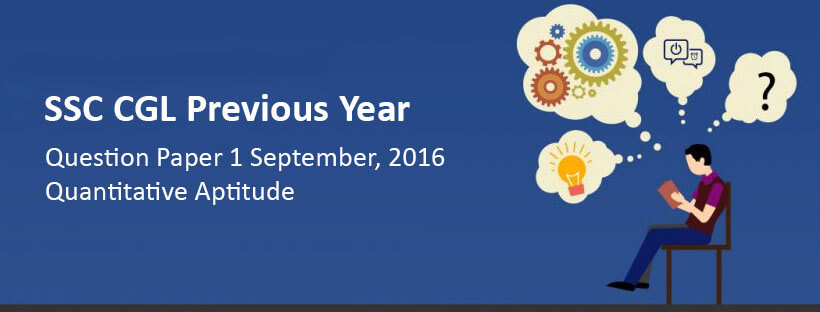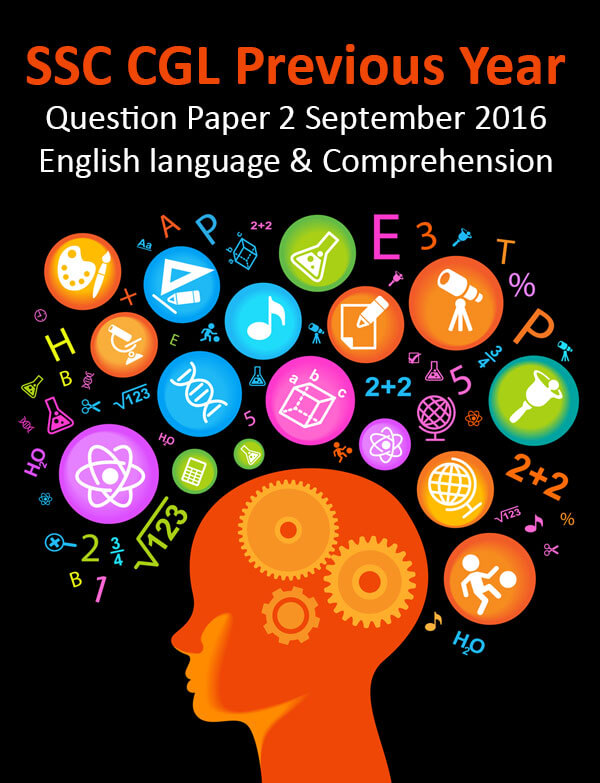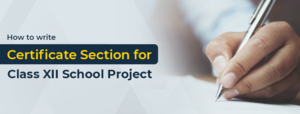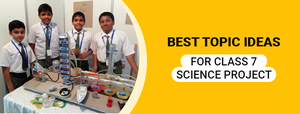SSC CGL Previous Year Question Paper 1 September 2016: Quant - Study24x7Default error msg

New to Study24x7 ?

# SSC CGL Previous Year Question Paper 1 September 2016: Quantitative Aptitude

Updated on 25 March 2020SSC Preparation Strategies & S
Updated on 25 March 2020Question 1. A and B together can finish a work in 30 days. They worked for it for 20 days and then B left the work. The remaining work was done by A alone in 20 days more. In how many days can A alone finish the work?

a. 48 days

b. 50 days

c. 54 days

d. 60 days

Question 2. The centroid of an equilateral triangle ABC is G. If AB is 6 cms, the length of AG is

a. √3 cm

b. 2√3 cm

c. 3√2 cm

d. 2√2 cmQuestion 3. A merchant changed his trade discount from 25% to 15%. This would increase selling price by

a. 3⅓%

b. 6⅙%

c. 13⅓%

d. 16⅓%

Question 4. If 177 is divided into 3 parts in the ratio 1/2 : 2/3: 4/5, then the second part is

a. 75

b. 45

c. 72

d. 60

Question 5. If percentage of profit made,when an article is sold for Rs.78, is twice as when it is sold for Rs.69, the cost price of the article is

a. Rs. 49

b. Rs. 51

c. Rs. 57

d. Rs. 60Question 7.Gautam travels 160 kms at 32 kmph and returns at 40 kmph. Then average speed is

a. 72 kmph

b. 71.11 kmph

c. 36 kmph

d. 35.55 kmph

Question 8.If x=3/2, then the value of 27x3-54x2+36x-11 is

a. 11⅜

b. 11⅝

c. 12⅜

d. 12⅝

Question 9.If a+b+c = 6 and ab+bc+ca = 11, then the value of bc(b+c) + ca(c+a) +ab(a+b) +3abc is

a. 33

b. 66

c. 55

d. 23

Question 10.If the angles of a triangle are in the ratio of 2:3:4, then the difference of the measure of greatest angle and smallest angle is

a. 20°

b. 30°

c. 40°

d. 50°

Question 11.In ΔABC,∠A = 90°, AD┴BC and AD = BD = 2 cm. The length of CD is

a. 3 cm

b. 3.5 cm

c. 3.2 cm

d. 2 cm

Question 12. If tan45 = cotθ,then the value of θ in radians in?

a. Π/4

b. Π/9

c. Π/2

d. Π/12

Question 13.(251 + 252+253+254+255) is divisible by

a. 23

b. 58

c. 124

d. 127

Question 14.The average of 12 numbers is 9. If each number is multiplied by 2 and added to 3, the average of the new set of numbers is

a. 9

b. 18

c. 21

d. 27Question 17.The perimeter of two similar triangles ABC and PQR are 36 cms and 24 cms respectively. If PQ = 10 cm then the length of AB is

a. 18 cm

b. 12 cm

c. 15 cm

d. 30 cm

Question 18.In a triangle ABC, AB = 8 cm, AC = 10 cm and∠B = 90°, then the area ofΔABC is

a. 49 sq.cm

b. 36 sq.cm

c. 25 sq.cm

d. 24 sq.cmQuestion 20.The compound interest on Rs. 64,000 for 3 years, compounded annually at 7.5% p.a. is

a. Rs. 14,400

b. Rs. 15,705

c. Rs. 15,507

d. Rs. 15,075

Question 21.The angles of elevation of the top of a temple, from the foot and the top of a building 30 m high, are 60° and 30° respectively. Then height of the temple is

a. 50 m

b. 43 m

c. 40 m

d. 45 m

Question 22.Study the pie-chart given and answer the following questions.If the miscellaneous charges are Rs. 6000, then the advertisement charges are

a. Rs.12000

b. Rs.27000

c. Rs.90000

d. Rs.25000

Question 23. The central angle of printing charge is x more than that of advertisement charge. Then the value of x is

a. 72°

b. 61.2°

c. 60°

d. 54.8°

Question 24. What should be the central angle of the sector 'cost of paper'?

a. 22.5°

b. 54.8°

c. 36°

d. 16°

Question 25. The ratio between royalty and binder's charges is

a. 5:6

b. 5:8

c. 6:5

d. 8:13

All The Best To All The SSC CGL 2020 Aspirants !

Write a comment...Trending ArticlesBy CBSE CLASS 8By CBSE CLASS 10By Edubabble LLPBy CBSE CLASS 7
Related PostsServicesNeed Some Help!Connect